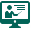Welcome to Comnet Group Inc.

## Artificial Intelligence and Deep Learning with TensorFlow

#### Course number: CGIAIDL40

AI and Deep Learning with TensorFlow is curated by industry professionals as per the industry requirements. You will master concepts such as SoftMax function, Autoencoder Neural Networks, and Restricted Boltzmann Machine (RBM), and work with libraries like Keras and TFLearn.

What you will learn:

Deep Learning in TensorFlow with Python Training will help you to become a Deep Learning Engineer. It will hone your skills by offering you comprehensive knowledge on Deep Learning in TensorFlow. It will also acquaint you with the required hands-on experience for solving real-time industry-based Deep Learning projects. During this course you will be trained by our expert instructors on:

• Deep Learning and TensorFlow Concepts
• Working with Convolutional Neural Network (CNN) and Recurrent Neural Network (RNN)
• Proficiency in Long short-term memory (LSTM)
• Implementing Keras, TFlearn, Autoencoders
• Implementing Restricted Boltz-mann Machine (RBM)
• Knowledge of Neural Networks & Natural Language Processing (NLP)
• Using Python with TensorFlow Libraries
• Perform Text Analytics
• Perform Text Processing
##### Prerequisites
• Basic programming knowledge in Python
##### Target Audience

This can be taken by all the professionals who are passionate about Deep Learning and want to go ahead and make their career as a Deep Learning Engineer. It is best suited for individuals who are:

• Developers aspiring to be a ‘Data Scientist’
• Analytics Managers who are leading a team of analysts
• Business Analysts who want to understand Deep Learning (ML) Techniques
• Information Architects who want to gain expertise in Predictive Analytics
• Analysts wanting to understand Data Science methodologies
##### Course Content
Introduction to Deep Learning

In this module, you’ll get an introduction to Deep Learning and understand how Deep Learning solves problems which Machine Learning cannot. Understand fundamentals of Machine Learning and relevant topics of Linear Algebra and Statistics.

Topics:

• Deep Learning: A revolution in Artificial Intelligence
• Limitations of Machine Learning
• What is Deep Learning?
• Advantage of Deep Learning over Machine Learning
• 3 Reasons to go for Deep Learning
• Real-Life use cases of Deep Learning
• Review of Machine Learning: Regression, Classification, Clustering, Reinforcement Learning, Underfitting and Overfitting, Optimization
Understanding Neural Networks with TensorFlow

In this module, you’ll get an introduction to Neural Networks and understand it’s working i.e. how it is trained, what are the various parameters considered for its training and the activation functions that are applied.

Topics:

• How Deep Learning Works
• Activation Functions Illustrate Perceptron
• Training a Perceptron
• Important Parameters of Perceptron
• What is TensorFlow?
• TensorFlow code-basics
• Graph Visualization
• Constants, Placeholders, Variables
• Creating a Model
• Step by Step – Use-Case Implementation
Deep Dive into Neural Networks with TensorFlow

In this module, you’ll understand backpropagation algorithm which is used for training Deep Networks. You will know how Deep Learning uses neural network and backpropagation to solve the problems that Machine Learning cannot.

Topics:

• Understand limitations of a Single Perceptron
• Understand Neural Networks in Detail
• Illustrate Multi-Layer Perceptron Backpropagation – Learning Algorithm
• Understand Backpropagation – Using Neural Network
• Example MLP Digit-Classifier using TensorFlow
• Tensor Board
Master Deep Networks

In this module, you’ll get started with the TensorFlow framework. You will understand how it works, its various data types & functionalities. In addition, you will create an image classification model.

Topics:

• Why Deep Networks
• Why Deep Networks give better accuracy
• Use-Case Implementation on SONAR dataset
• Understand How Deep Network Works
• How Backpropagation Works
• Illustrate Forward pass, Backward pass
• Different variants of Gradient Descent
• Types of Deep Networks
Convolutional Neural Networks (CNN)

In this module, you’ll understand convolutional neural networks and its applications. You will learn the working of CNN, and create a CNN model to solve a problem.

Topics:

• Introduction to CNNs
• CNNs Application
• Architecture of a CNN
• Convolution and Pooling layers in a CNN
• Understanding and Visualizing a CNN
Recurrent Neural Networks (RNN)

In this module, you’ll understand Recurrent Neural Networks and its applications. You will understand the working of RNN, how LSTM are used in RNN, what is Recursive Neural Tensor Network Theory, and finally you will learn to create an RNN model.

Topics:

• Introduction to RNN Model
• Application use cases of RNN
• Modelling sequences
• Training RNNs with Backpropagation
• Long Short-Term memory (LSTM)
• Recursive Neural Tensor Network Theory
• Recurrent Neural Network Model
Restricted Boltzmann Machine (RBM) and Autoencoders

In this module, you’ll understand RBM & Autoencoders along with their applications. You will understand the working of RBM & Autoencoders, illustrate Collaborative Filtering using RBM and understand what Deep Belief Networks are.

Topics:

• Restricted Boltzmann Machine
• Applications of RBM
• Collaborative Filtering with RBM
• Introduction to Autoencoders
• Autoencoders applications
• Understanding Autoencoders
Keras API

In this module, you’ll understand how to use Keras API for implementing Neural Networks. The goal is to understand various functions and features that Keras provides to make the task of neural network implementation easy.

Topics:

• Define Keras
• How to compose Models in Keras
• Sequential Composition
• Functional Composition
• Predefined Neural Network Layers
• What is Batch Normalization
• Customizing the Training Process Using Tensor Board with Keras
• Use-Case Implementation with Keras
TFLearn API

In this module, you’ll understand how to use TFLearn API for implementing Neural Networks. The goal is to understand various functions and features that TFLearn provides to make the task of neural network implementation easy.

Topics:

• Define TFLearn
• Composing Models in TFLearn
• Sequential Composition
• Functional Composition
• Predefined Neural Network Layers
• What is Batch NormalizationLive Online